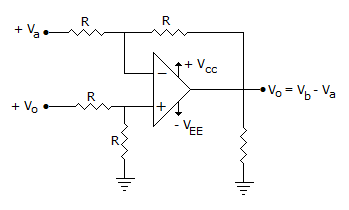# Electronics and Communication Engineering - Analog Electronics

36.

An ideal op-amp can amplify dc signals

 A. True B. False

Explanation:

No answer description available for this question. Let us discuss.

37.

Consider the following statements :
The lower cut off frequencies of an RC coupled amplifier depend on

1. input and output coupling capacitor
2. emitter by pass capacitors
3. junction capacitances
Which of the above are correct?

 A. 1 only B. 2 only C. 1 and 2 D. 2 and 3

Explanation:

No answer description available for this question. Let us discuss.

38.

Assertion (A): In a circuit using FET, gate is approximately at dc ground

Reason (R): Self bias stabilizes the quiescent operating point against changes in JFET parameters

 A. Both A and R are correct and R is correct explanation for A B. Both A and R are correct but R is not correct explanation for A C. A is correct R is wrong D. A is wrong R is correct

Explanation:

No answer description available for this question. Let us discuss.

39.

The Darlington pair consists of the following two stages

 A. CE and CC B. both CE C. both CC D. CE and CB

Explanation:

No answer description available for this question. Let us discuss.

40.

Figure representsA. Adder B. Multiplier C. Subtractor D. Log Amplifier